Next: Ideal Points and Up: Important concepts from projective Previous: Projective Transformations

# Projective Invariants

A significant property of the projective transformations is that certain measurements are invariant under these transformations. The usefulness of invariants in geometrical problems is probably most familiar in Euclidian geometry. In this case, the transformations are rotation and translation and the most important invariants are distance and angle - consequently, distances and angles are key concepts in a Euclidian analysis. For projective transformations, the most fundamental invariant is called the cross-ratio. According to [Mundy 1992], ``It seems likely that all invariant properties of a geometric configuration can ultimately be interpreted in terms of some number of cross-ratio constructions'' (our italics).

The cross-ratio can be defined for four collinear points or four concurrent lines, Figure 2. The cross-ratio of the four points in Figure 2a is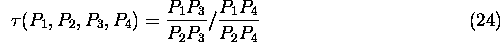where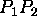is the distance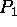to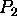. The cross-ratio of the four lines in Figure 2b is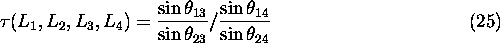where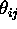is the angle between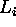and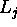.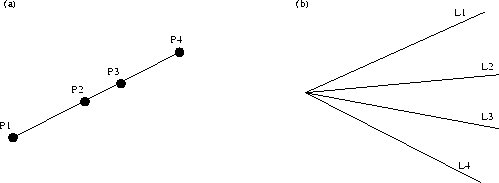Figure: The cross-ratio is defined for a construction of four collinear points or four concurrent lines as described in the text.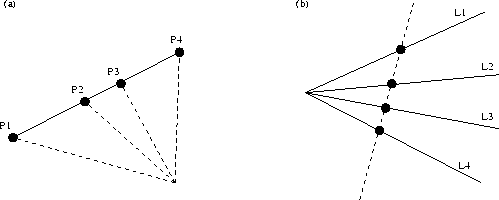Figure: The points and lines in Figure 2 are used as the basis for these constructions. The cross-ratio provides an interesting link between the original points (lines) and the constructed lines (points) as described in the text.

A set of points such as those in Figure 2a can be associated with a construction of lines as shown in Figure 3a. A useful fact is that the cross-ratio of the original four points is equal to the cross-ratio of the constructed lines. Similarly, the lines in Figure 2b can be associated with a construction of points as shown in Figure 3b. The cross-ratio of the original four lines is equal to the cross-ratio of the four constructed points.

For a specific example of the invariance of the cross-ratio, consider four image points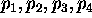which correspond to four points in a scene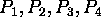. In this case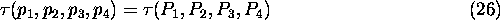One problem arises though. In general a projective transformation can cause certain permutations of the four collinear points and, depending on the particular permutation, this can change the cross-ratio. If the image points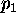and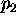in Equation (26) are permuted for example, then,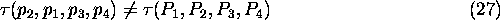This can be dealt with by using the j-invariant instead of the cross-ratio to provide the invariant measure. The j-invariant is defined in terms of the cross-ratio,The j-invariant has a fixed value regardless of the ordering of the points so,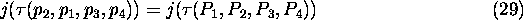which can be compared with Equation (27).Next: Ideal Points and Up: Important concepts from projective Previous: Projective Transformations

Bob Fisher
Fri Nov 7 12:08:26 GMT 1997